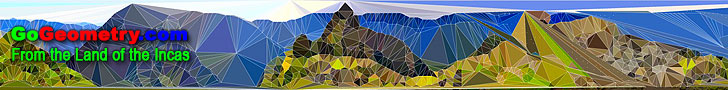# Welcome to GoGeometry: Recent additions (Page 2), Online LearningGo Geometry from the Land of the Incas - Recent Additions (Page 2 of 50)Geometry Problem 989. Arbelos, Semicircles, Diameter, Perpendicular, 90 Degree, Common External Tangent, Rectangle, Midpoint of Arc. Geometry Problem 986. Triangle, Median, Midpoint, Equal Angles, 45 Degrees. Geometry Problem 988. Right Triangle, Cevians, Angles, 30 Degrees, Triple Angle. Geometry Art 901 - 910. Bicentric Quadrilateral, Incircle, Circumcircle, Circumscribed, Inscribed, Perpendicular. Kaleidoscope: Geometry Problem Art 901 - 910. iPad apps: Kaleidoscope X. Geometry Problem 987. Triangle, Circumcircle, Incenter, Chord, Parallel, Circle, Tangent. GeoGebra, HTML5 Animation for Tablets (iPad, Nexus). Geometry Problem 985. Circle, Radius, Perpendicular, Concentric Circles, Congruence, Triangle. Geometry Problem 984. Triangle, Circumcircle, Altitudes, Midpoint, Collinear Points. Geometry Problem 983. Regular Hexagon, A point and six triangles, Areas. Geometry Problem 982. Triangle, Excenters, Excentral Triangle, Circumcenter, Area, Hexagon. Geometry Problem 981. Triangle, Concurrent Cevians, Midpoints, Area, Hexagon. Geometry Problems 971 - 980. Equilateral Triangle, Rectangle, Circle, Parallel, Reflection, Concurrency, Parallelogram, Orthocenter, Circumcenter. Geometry Problem 980. Equilateral Triangle, Vertices, Three Parallel, Equal Circles, Construction. Geometry Problem 979. Triangle, Cevian, Angle Bisector, Concurrency, 90 Degrees, Perpendicular. Geometry Problem 978. Parallelogram, Diagonal, Transversal, Similarity, Metric Relations. Geometry Problem 977. Acute Triangle, Circumcenter, Orthocenter, Distance, Circumradius, Altitude, Metric Relations. Geometry Problem 976. Isosceles Right Triangle, 45 Degrees, Incenter, Angle Bisector, Hypotenuse. Geometry Problem 975. Isosceles Right Triangle, 45 Degrees, Angle Bisector, Hypotenuse. Geometry Problem 974. 'Begonia Theorem', Cevian Triangle, Reflection of a point in a line, Concurrency of Lines. GeoGebra, HTML5 Animation for Tablets. Geometry Problem 973. Three Parallel, Square, Vertices, Distance Between Two Parallel Lines, Side, Metric Relations. Geometry Problem 972. Equilateral Triangle, Vertices, Three Parallel, Equal Circles, Perpendicular Bisector. Australia's first Peruvian art exhibition to open in Canberra this year. Gold of the Incas. The exhibition, staged to mark the 50th anniversary of Australian-Peruvian diplomatic relations, runs until 21 April, 2014 Geometry Problem 971. Equilateral Triangle, Rectangle, Common Vertex, Sum of Right Triangles Areas. Geometry Problem 970. Equilateral Triangle, Circumcircle, Secant, Metric Relations. Geometry Problem 969. Equilateral Triangle, Rectangle, Common Vertex, Right Triangles Areas. Albrecht Durer: Melencolia I (1514), Female Figure and Golden Rectangles The melancholic temperament is personified by the female figure. Geometry Problem 968. Equilateral Triangle, Rectangle, Common Vertex, Midpoint. Geometry Problem 967. Three Equilateral Triangles, Midpoints. GeoGebra, HTML5 Animation for Tablets (iPad, Nexus). Geometry Problem 966. Triangle, Cevians, Midpoint, Circumcircle, Circle, Tangent Line, Collinear Points. Geometry Problem 965. Triangle, Cevians, Midpoint, Circumcircle, Circle, Tangent Line, Metric Relations. Geometry Problem 964. Right Triangle, Cevians, Angles, 10, 20, 30 Degrees. Geometry Problem 963. Right Triangle, 30-60-90 Degrees, Angle Bisectors, Metric Relations. Geometry Problem 962. Triangle, Two Cevians, Areas, Equal Products. Geometry Problem 961. Quadrilateral, Trisection, Sides, Sum of Areas. Math Quote: Archimedes. Word cloud. Go to Page: Previous | 1 | 10 | 20 | 30 | 40 | 50 | Next
 Home | GoGeometry Education | Geometry | Search | Email | By Antonio Gutierrez Last updated Feb 21, 2015